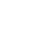Making functions using foldl in PureScript

Published 4 years ago
Updated 3 years ago

foldl gives us a lot of flexibility when working with lists and arrays. You can use foldl to create new functions to manipulate data exactly how you need to.

As long as the types match, foldl will accept many functions to apply the specific behavior you need. In this lesson, we’ll look at how to create a map and filter using foldl.

Instructor: [00:00] Let's play with making functions out of foldL. We've got our own version of foldL, and we've called it foldltick. Let's change the function to a times, so 01 * 2 * 3 * 4 is 0We'll do a minus, 01- 2 - 3 - 4. That'll give us -10. That works as we expect a foldL to work. Let's put that back to plus.

[00:23] Now let's create our own functions out of foldltick. First, we'll try and make a map. We'll call that maptick, and that has a type of forall a b. It takes a function of a -> b, a list of a, and returns us a list of b. On the next line, we'll do maptick F Xs, and that equals fold tick, a lambda function of acc, and X.

[00:50] Then we have acc appended to F(X), which is cons with nil. Then we pass in nil and Xs. We'll test this out. We'll do log show, and we'll put maptick with a function of _ + 1. We'll copy mine. Let's put it here. That'll return us 2, 3, 4, 5, and nil.

[01:13] The trick is, inside of here, we're appending acc to the cons of F X. We're actually running our function on each element, with the F. When it first come through, 1 would be 1 + 1, so that'd be 2. It'd accumulate and it'd keep going. It would be 1 + 1 is 2, 2 + 1 is 3, 3 + 1 is 4, and so on.

[01:36] We're using forall to recurse over our list. Through each pass, we're running our function against each element and accumulating them altogether into a single list.

[01:44] Next, let's make a filter with foldL. We'll start by writing filter tick, and it has a type of forall a b, and that takes a function from a -> Boolean -- a list of a and returns us a list of a. On the next line, we'll do filter tick F Xs. That equals a foldL tick.

[02:04] On the next line, it'll be our lambda function with acc and X. Then we'll do acc and append it to if F X, then we'll cons X onto nil. Otherwise, we'll return nil. Then we'll pass nil and Xs to foldltick. Let's quickly try that out.

[02:20] We'll do filter tick, and it takes a function of _ > 1, and we'll pass it myIntList. As expected, it returns us a list of 2, 3, 4, and nil. Let's try it out with greater than 2. Yep, that returns us 3, 4, and nil. We're filtering out all the values that aren't greater than 2.

[02:38] You should know that filter and map are really similar. Where they differ is inside the lambda function that we pass to foldltick. In map, we run F on X. It's a function of a -> b. In filter, we're doing a function from a -> Boolean. To do that, we do an ifThen and checking, if we pass X to our function, does that return true or false? For this occurrence, is X greater than 2.

[03:01] The great thing about foldL is it will take a function from b -> a -> b, but it doesn't really mind what you do inside that function, as long the type matches. That's how we're able to create a map and a filter using foldL.

[03:13] Let's make one more function. This time, we'll make a reverse. We'll do reverse tick, and that has a type of forall a b -- list a and returns us a list a. On the next line, we'll do reverse tick Xs. That equals foldL tick, and that's got a lambda function with the inputs of acc and X. It will do cons X and acc. Then we do nil and Xs.

[03:40] Let's quickly try that out. We'll copy this, and we'll change filter to reverse tick. We'll remove this function out. If we look at our output, we've got 4, 3, 2, 1, and nil. The trick to reverse is inside our lambda function. Here, we've got cons X acc. We'll quickly demonstrate that.

[04:00] We'll write it out cons, X, and acc. In that case, when it first comes around, X is 4. What's happening is we put an X first onto cont, and then acc, because every time there is a recursion inside foldL, we cons X to the beginning of our list. The result with that is we accumulate a new list, which starts with 4, 3, 2, 1, then nil.

[04:22] There you have it, an example of how we were able to use foldL to create totally new functions.Robert Pearce
~ 4 years ago

I see filter' and reverse' have forall a b, but I don't see b used in the type definition. Was this done on purpose?